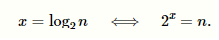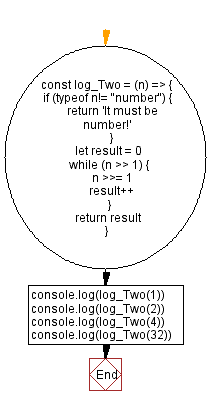# JavaScript - Binary logarithm using bitwise operators

## JavaScript Bit Manipulation: Exercise-8 with Solution

Write a JavaScript program to calculate the binary logarithm (log2n) using bitwise operators.

From handwiki.org -
Binary logarithm:
In mathematics, the binary logarithm (log2n) is the power to which the number 2 must be raised to obtain the value n. That is, for any real number x,For example, the binary logarithm of 1 is 0, the binary logarithm of 2 is 1, the binary logarithm of 4 is 2, and the binary logarithm of 32 is 5.
The binary logarithm is the logarithm to the base 2 and is the inverse function of the power of two function. As well as log2, an alternative notation for the binary logarithm is lb (the notation preferred by ISO 31-11 and ISO 80000-2).

Test Data:
(1) -> 0
(2) -> 1
(4) -> 2
(32) -> 5

Sample Solution:

JavaScript Code:

``````const log_Two = (n) => {
if (typeof n!= "number") {
return 'It must be number!'
}
let result = 0
while (n >> 1) {
n >>= 1
result++
}
return result
}
console.log(log_Two(1))
console.log(log_Two(2))
console.log(log_Two(4))
console.log(log_Two(32))
```
```

Sample Output:

```0
1
2
5
```

Flowchart:Live Demo:

See the Pen javascript-bit-manipulation-exercise-8 by w3resource (@w3resource) on CodePen.

* To run the code mouse over on Result panel and click on 'RERUN' button.*

Improve this sample solution and post your code through Disqus

What is the difficulty level of this exercise?

Test your Programming skills with w3resource's quiz.

﻿